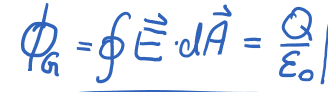# Gauss' Law: Understand How to Calculate Flux

• sayuri2009

#### sayuri2009

Homework Statement
Gauss Law for flux
Relevant Equations
flux = Integration E ds = Q / e0
Hello everybody

To calculate the flux for the electric field I need the gauss law. There are two formula one with the integration over some area and the other is Q/e0. When do I have to use which one?sayuri2009 said:
Homework Statement:: Gauss Law for flux
Relevant Equations:: flux = Integration E ds = Q / e0

Hello everybody

To calculate the flux for the electric field I need the gauss law. There are two formula one with the integration over some area and the other is Q/e0. When do I have to use which one?
View attachment 275550
I depends on what is given to you. If you know the electric field, you do the integral to find the flux. If you know the charge enclosed by the surface, you divide that charge by ε0 and you have the flux through the surface.

•sayuri2009